# Famous theorems in plane geometry and geometry in space

## Pythagorean Theorem

In a rectangular triangle, the square of the hypotenuse is equal to the length of the squares of the cathetes.ΔABC rectangular triangle having the measure of the A angle equals 90°

Then we have:
BC2=AB2 + AC2

## Reciprocal of Pythagorean Theorem

If in a triangle the square of the length of one side is equal to the sum of the squares of the lengths of the other two sides then the triangle is rectangular.

If in ΔABC we have BC2=AB2+AC2 then m(A)=90°.

## The theorem of three perpendiculars

Let  α a geometric plan, (d) a right included in the geometric plan α and A ∉ α, O ∈ α, O ∉ d. If AO⊥α, OB ⊥d, then AB⊥d.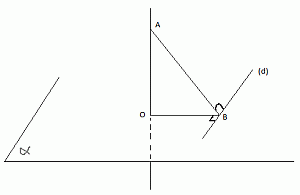The reciproc 1. Let α a geometric plan, (d) a right line included in the plan α, A ∉ α, O ∈ α, O ∉ d, B∈d. If AOα and ABd, then OBd.

The reciproc 2. Let α a geometric plan, (d) a right line included in the plan α, A ∉ α, O ∈ α, O ∉ d, B∈d. If AOOB, ABd and OBd, then AOd.

## Ceva's Theorem

Let be the triangle ABC and the points A'∈(BC), B'∈(CA), C'∈(AB). If the right lines AA', BB' and CC' are concurrent (htey have a common point), then we have: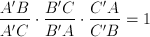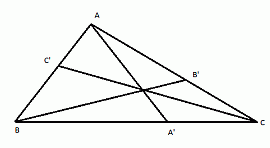The reciprocally of Ceva's Theorem. Let be the triangle ABC and the points A'∈(BC), B'∈(CA), C'∈(AB). If the bellow relation is truethen the right laines AA', BB' and CC' are concurrent (have a common point).

## Van Aubel relationship

Let the triangle ABC and the points A'(BC), C∈(AC'), B∈(AB'). If the right lines AA', CB' È™i BC' are concurrent into a point P, then exists the relation: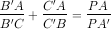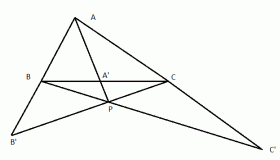## Menelaos Theorem

Let the triangle ABC and A', B' and C' three points such as  A'∈(BC), B'∈(CA), C'∈(AB). If the points A', B' and C' are collinear, then: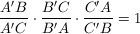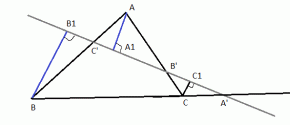Menelaos reciprocically. Let the triangle ABC and the points A'∈(BC), B'∈(CA), C'∈(AB) such as two of the points A', B', C' are situated on two sides of the triangle, and the 3th on the extension of the 3th side of the triangle. If it is true thatthen the points A', B' and C' are collinear.

## Theorem parallels unequidistant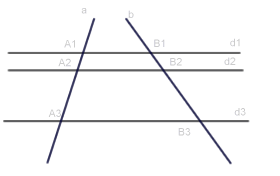Several parallel lines on two secant  determined some proportionate segments. Let d1 || d2 || d3 coplanare with the right lines a and b and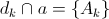,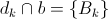,

whatever k ∈ {1, 2, 3}. Then: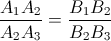## Theorem bisecting

In a triangle the bisector of the angle divides the outer face of a side opposite to the side that is proportional to the segments that form the angle.In the triangle ABC let the bisect (AD of an angle BAC, with D[BC]. Then we have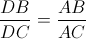## Thales' Theorem

Let the triangle ABC and the line d || BC, D and E intersections point of the line d with the sides AB and AC. Then: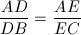orKeywords: Theorem, Pythagoras, geometry, Menelaus, Ceva's Theorem, Theorem of the 3 perpendicular relationship Van Aubel

### Forum

Here you can discuss about mathematic, about algebra, geometry, trigonometry.

It is not mandatory to be logged in on this forum but it is nice to have an account. You can ask about mathematics just with your name and your email.

This maths forum is one of the easiest forums to use it.

>> Go to Math Forum